• +91 9971497814
• info@interviewmaterial.com

# Chapter 9 Sequences and Series Ex-9.1 Interview Questions Answers

### Related Subjects

Question 1 : Write the first five terms of each of the sequences in Exercises 1 to 6 whose nth terms are:
an = n (n + 2)

Given,

nth termof a sequence an = n (n + 2)

On substituting n =1, 2, 3, 4, and 5, we get the first five terms

a1 =1(1 + 2) = 3

a2 =2(2 + 2) = 8

a3 =3(3 + 2) = 15

a4 =4(4 + 2) = 24

a5 =5(5 + 2) = 35

Hence, the requiredterms are 3, 8, 15, 24, and 35.

Question 2 :

an = n/n+1

Given nth term,an = n/n+1

On substituting n =1, 2, 3, 4, 5, we get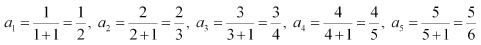Hence, the requiredterms are 1/2, 2/3, 3/4, 4/5 and 5/6.

Question 3 :

an = 2n

Given nth term, an =2n

On substituting n =1, 2, 3, 4, 5, we get

a1 = 21 =2

a2 = 22 =4

a3 = 23 =8

a4 = 24 =16

a5 = 25 =32

Hence, the requiredterms are 2, 4, 8, 16, and 32.

Question 4 :

an = (2n – 3)/6

Given nth term, an =(2n – 3)/6

On substituting =1, 2, 3, 4, 5, we get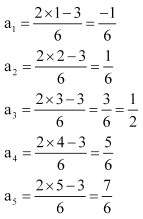Hence, the requiredterms are -1/6, 1/6, 1/2, 5/6 and 7/6..

Question 5 :

an = (-1)n-1 5n+1

Given nth term,an = (-1)n-1 5n+1

On substituting =1, 2, 3, 4, 5, we get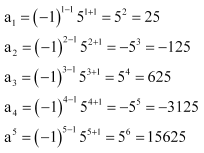Hence, the requiredterms are 25, –125, 625, –3125, and 15625.

Question 6 :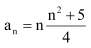On substituting n =1, 2, 3, 4, 5, we get first 5 terms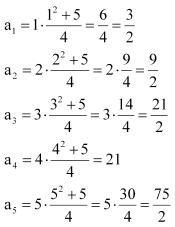Hence, the requiredterms are 3/2, 9/2, 21/2, 21 and 75/2.

Question 7 :

Find the indicated terms in each of the sequences in Exercises 7 to 10whose nth terms are:

an = 4n – 3; a17, a24

Given,

nth term of the sequence is an = 4n – 3

On substituting n =17, we get

a17 =4(17) – 3 = 68 – 3 = 65

Next, onsubstituting n = 24, we get

a24 =4(24) – 3 = 96 – 3 = 93

Question 8 :

Find the indicated terms in each of the sequences in Exercises 7 to 10whose nth terms are:

an = n2/2n ; a7

Given,

nth term of the sequence is an = n2/2n

Now, onsubstituting n = 7, we get

a7 = 72/27 =49/ 128

Question 9 : Find the indicated terms in each of the sequences in Exercises 7 to 10whose n_th terms are:

an = (-1)n-1 n3; a9

Given,

nth term of the sequence is an = (-1)n-1 n3

On substituting n =9, we get

a9 =(-1)9-1 (9)3 = 1 x 729 = 729

Question 10 : Find the indicated terms in each of the sequences in Exercises 7 to 10whose n_th terms are: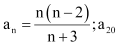On substituting n =20, we get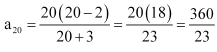Todays Deals### Chapter 9 Sequences and Series Ex-9.1 Contributorskrishan

Name:
Email:

# Latest News# 9000 interview questions in different categories